# RobertNachbar/CompartmentalModeling

Build, visualize, analyze, and simulate compartmental models

Contributed By: rnachbar@wolfram.com  |  Robert Nachbar

Compartmental models are a convenient way to describe and analyse processes that involve the movement of items from one place or state to another. The items can be people, money, molecules, ideas, …. The compartmental model itself is just a representation of the information or data, and can be converted into other mathematical representations, such as differential equations, for analysis. Compartmental models are used frequently in the fields of chemical reactions, systems biology, ecology, epidemiology, pharmacokinetics, and many more.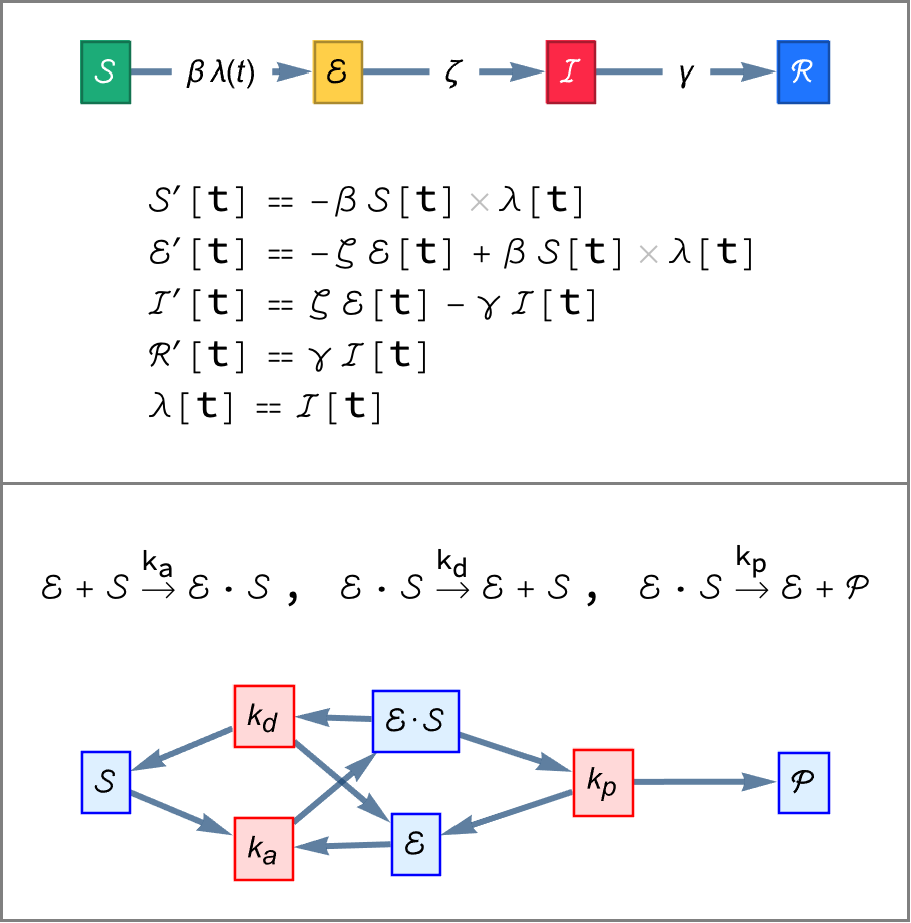## Installation Instructions

To install this paclet in your Wolfram Language environment, evaluate this code:
PacletInstall[ResourceObject["RobertNachbar/CompartmentalModeling"]]

## Details

The CompartmentalModeling paclet is intended to support compartment models in general.
The Transition is the fundemental object from which compartmental models are built in Wolfram Language; it describes the movement of items from one place to another or the change of state of one item to another.
Domain-specific functionality is provided for dynamic transmission models in epidemiology and for systems biology models (including chemical reaction kinetics); support for pharmacokinetics is planned.
Version 12.1 or newer of the Wolfram Language is required.

## Examples

### Basic Examples (3)

Write a transition for the infection of a susceptible individual for an SIR epidemiology model:

 In:=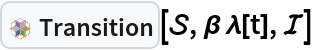Out=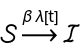Write a transition for a reaction for a reaction network model:

 In:=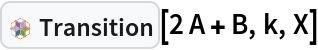Out=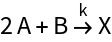Write a transition for the absorption of a drug from the gut into the blood stream for a pharmacokinetics model:

 In:=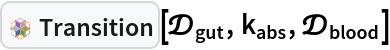Out=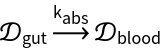Generate the reactions for an enzyme catalyzed reaction:

 In:=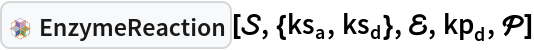Out=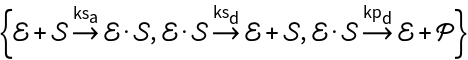Draw the compartmental model graph of a simple reaction network:

 In:=Out=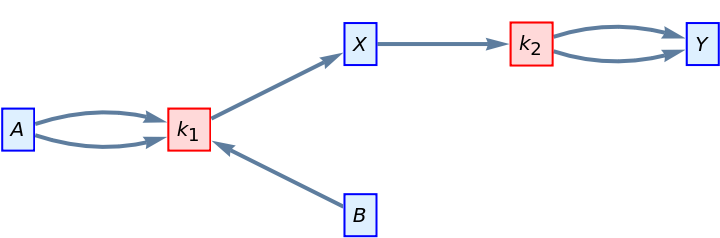Draw the compartmental model graph of an SEIR epidemiology model:

 In:=Out=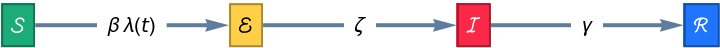Draw the compartmental model graph of a pharmacokinetics model:

 In:=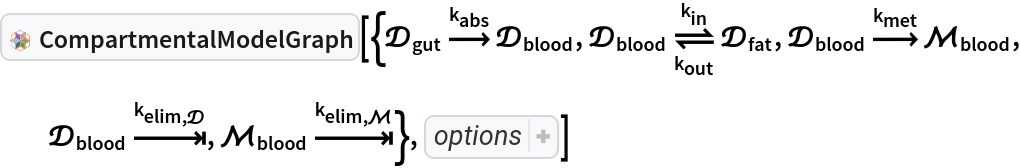Out=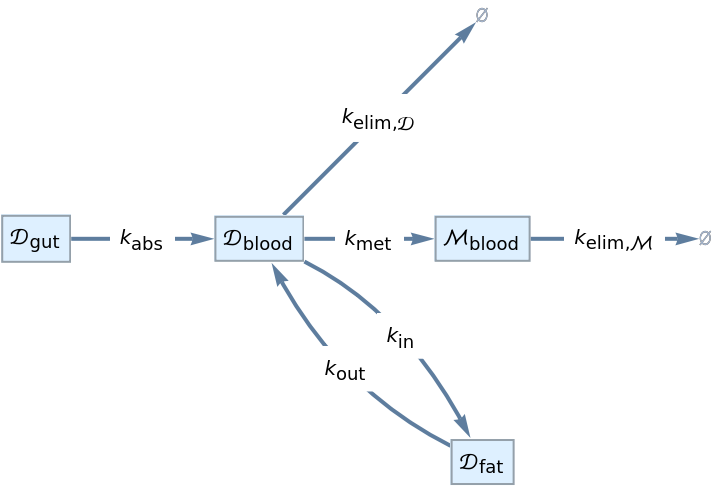Generate the Michaelis-Menten kinetic law for an enzyme catalyzed reaction with a competitive inhibitor:

 In:=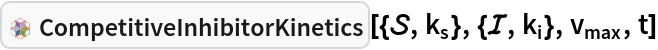Out=### Scope (2)

Add transitions to an SEIR epidemiology model for vital demographics:

 In:=Out=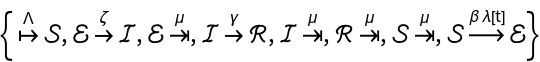In:=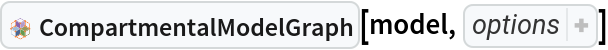Out=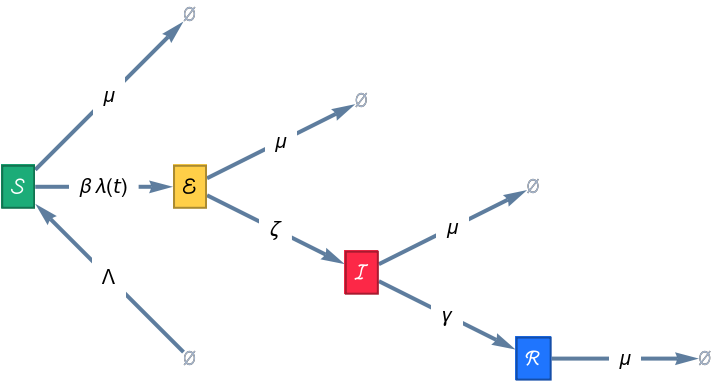Compute the ODEs, stoichiometric matrix, reaction rates, and other model data for a reaction network model:

 In:=Out=Compute the ODEs, force of infection, and other model data for an age-stratified SEIR epidemiology model:

 In:=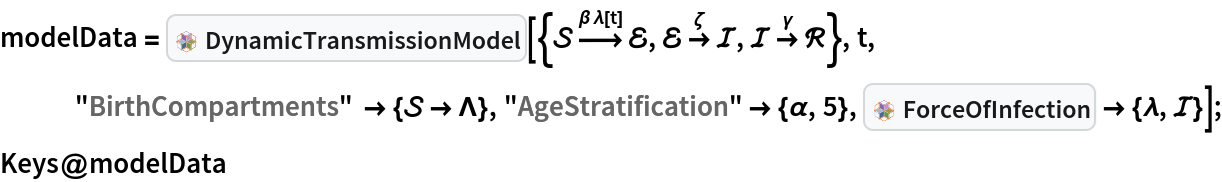Out=In:=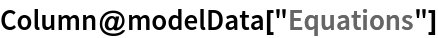Out=In:=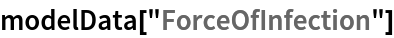Out=